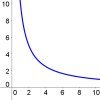#### You may also like### Complex Partial Fractions

To break down an algebraic fraction into partial fractions in which all the denominators are linear and all the numerators are constants you sometimes need complex numbers.### Agile Algebra

Observe symmetries and engage the power of substitution to solve complicated equations.### Graphs of Changing Areas

Use graphs to gain insights into an area and perimeter problem, or use your knowledge of area and perimeter to gain insights into the graphs...

# Steve's Mapping

### Why work on this question?

This month's NRICH site has been inspired by the way teachers at Kingsfield School in Bristol work with their students. Following an introduction to a potentially rich starting point, a considerable proportion of the lesson time at Kingsfield is dedicated to working on questions, ideas and conjectures generated by students.

Working on this question will encourage students to work together, discuss ideas, develop conjectures, suggest new lines of enquiry and solve problems.

### Here are the sort of questions that might emerge:

What affects the direction and steepness of a graph?
Can I tell from a function where its graph will cross the axes?
Which functions give straight lines, and which give curves?
When will two quadratic functions intersect?
Can I tell from a quadratic function where its graph has a turning point?

The interactivity could be used as a starting point to encourage students to make conjectures about functions and graphs, in a similar way to how students at Kingsfield School are introduced to the topic.

Read the article Kingsfield School - Building on Rich Starting Points, which has links to a description of a Kingsfield teacher's first lesson on functions and graphs, and a video showing how these ideas are put into practice in the classroom.

For teachers who want to create their own alternatives to Steve's Mapping for use in the classroom, here is an introductory video explaining how to build, load and save your own examples.

To read more about the Number Plumber, visit Grumplet's blog where you can comment on how you have used the number plumber and share links to files you have created. We are continuing to develop this resource so your feedback and ideas will be very useful.

### Key questions

What do you think these function machines do?
What will happen if we input 5? 13? 100? 0.7? ...

Is it possible to get the same output from both machines using the same input number? Is there more than one way?

What other questions could we ask?
Can you make any predictions about what might happen when we change the function machines?
What's the same? What is different?
Can you explain?

### Possible extension

Students can create and investigate their own Rational Functions using the NRICH Number Plumber